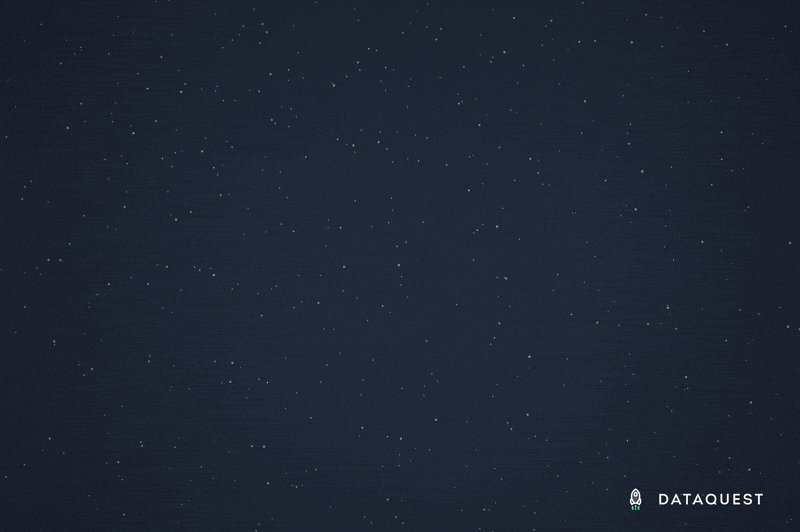# New Python Statistics Course: Conditional Probability

Published: October 31, 2019

Take your data science and statistics knowledge to the next level with the latest addition to our data science course offerings: Conditional Probability.In this course, you'll learn about the basics of conditional probability and then dig into more advanced concepts like Bayes's Theorem and Naive Bayes algorithms. As you learn, you'll be using your Python skills to put theory into practice and build a working knowledge of these critical statistics concepts.

## What's Covered in Conditional Probability?

Conditional Probability is an area of probability theory that's concerned with — as the name suggests — measuring the probability of a particular event occurring based on certain conditions.

In this course, which builds off of the Probability Fundamentals course that precedes it, we'll start with some lessons on foundational concepts like the conditional probability formula, the multiplication rule, statistical dependence and independence, and more.

From there, we'll look at Bayes' Theorem and how it can be used to calculate probabilities. We'll examine prior and posterior probability distributions. Then we'll dig in and apply some of these statistical concepts by learning about the Naive Bayes algorithm, a common statistical tool employed by data scientists.

Finally, you'll put all your new knowledge into practice in a new guided project that challenges you to build an SMS spam filter using a data set of over 5,000 messages by employing a Naive Bayes algorithm.

By the end of the course, you'll feel comfortable assigning probabilities to events based on conditions using the rules of conditional probability. You'll know when these events have statistical dependence (or not) on other events. You'll be able to assign probabilities based on prior knowledge using Bayes's theorem.

And of course you'll have built a cool SMS spam filter that makes use of a Naive Bayes algorithm (and your Python programming skills)!

## Why Do I Need to Know This?

Conditional probability is an important area of statistics that comes up pretty frequently in data analysis and data science work. Understanding it is important for making sure that your analysis is on firm statistical footing, and you're not drawing the wrong conclusions from your data.

Practically speaking, questions on Bayes's theorem and the Naive Bayes algorithm specifically are fairly common in data science job interviews. You might be asked, for example, to explain what's going on "under the hood" with the Naive Bayes algorithm. Understanding how it works — which we cover in this course — helps you demonstrate that you're not just copy-pasting from GitHub, and that you really understand the math that underlies your analysis.

So why wait? Start learning conditional probability today:

#### Charlie Custer

Charlie is a student of data science, and also a content marketer at Dataquest. In his free time, he's learning to mountain bike and making videos about it.

Tags

announcements, course launches, naive-bayes, probability, statistics

## Learner Spotlight: Data Engineer Student Veena Sanjeeve

Learner Spotlight: Data Engineer Student Veena Sanjeeve

## How to Plot a Bar Graph in Matplotlib: The Easy Way

How to Plot a Bar Graph in Matplotlib: The Easy Way

## Achieve your data career goals with confidence.

Looking for something specific? Browse our course catalogue.